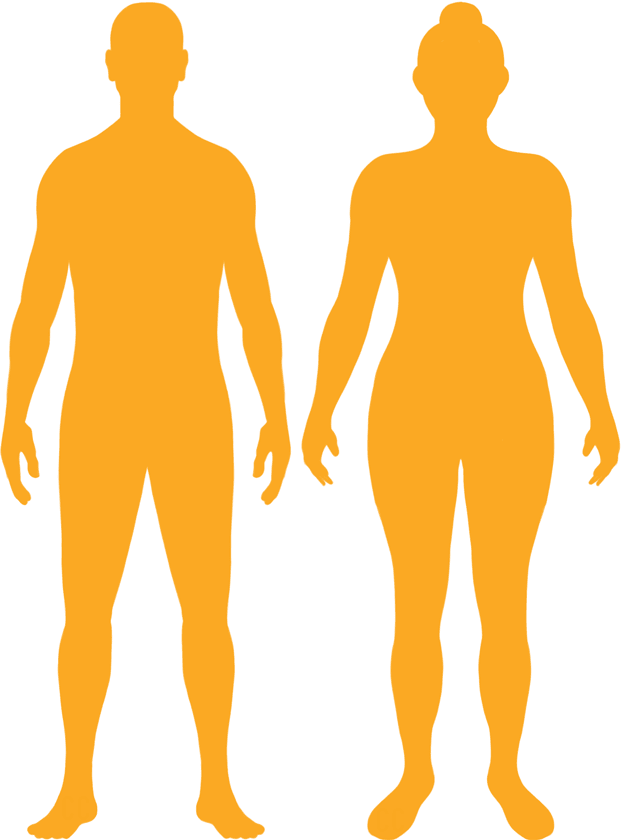# BMI Calculator

Result for 5'1" and 151 lbs.
Change Here.28.53

### Overweight BMI

At 5'1" and 151 Pounds your BMI is 28.53

### BMI Scale

 Under Normal Over Obese
-19 lbs
to reach a
normal BMI classification.
##### Normal BMI Ends:
132.3 lbs
+8 lbs
until you reach an obese BMI classification.
158.8 lbs

### BMI Grading Table2 for Height 5'1"

 Weight Weight Class Below 97.9 lbs Underweight 97.9 lbs - 132.3 lbs Normal Weight 132.3 lbs - 158.8 lbs Overweight 158.8 lbs and Above Obese

### Weight Loss

If you were seeking a normal BMI weight of 132.3lbs, and you lost two pounds a week4, then you could reach a normal BMI in
9.5 Weeks

### Overweight BMI Silhouette### Ideal Weight for a 5 foot 1 Male or Female2

The ideal weight range using the BMI for a male or female with a height of 5'1" is

### Between

97.9 lbs

and
132.3 lbs
How to Calculate BMI3 for 5'1" and 151 lbs
(5 x 12) + 1 = 61
(151 / (61 x 61)) x 703
= 28.528083848428
5 = foot | 1 = inch | 151 = weight lbs

### Conversion

• 5 foot 1 = 61 inches
• 5 foot 1 = 154.94cm
• 151 pounds = 10 stone 11 pounds
• 151 pounds = 68.49 kg
• 151 pounds = 68492.45 grams

### 5'1" and 151 lbs Summary

What is the BMI for a 5'1" and 151 lbs female? 28.53 BMI, Overweight.
What is the BMI for a 5'1" and 151 lbs male? 28.53 BMI, Overweight.
What is the ideal weight for a 5'1" female? Between: 97.9lbs and 132.3lbs
What is the ideal weight for a 5'1" male? Between: 97.9lbs and 132.3lbs
If I am 5ft 1in and weigh 151 lbs, is that a good weight for my height? Under the BMI classification, 151 lbs is classed as being Overweight.